Function Repository Resource:

# MultinormalKLDivergence

Compute the Kullback–Leibler divergence between two multinormal distributions

Contributed by: Seth J. Chandler
 ResourceFunction["MultinormalKLDivergence"][dist1,dist2] computes the Kullback–Leibler divergence between two multinormal distributions.

## Details

MultinormalKLDivergence will work with a univariate NormalDistribution or a bivariate BinormalDistribution. It treats them as special cases of the more general MultinormalDistribution.

## Examples

### Basic Examples (2)

Compute the Kullback–Leibler divergence for two multinormal distributions:

 In:=Out=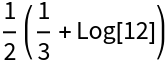Plot how the Kullback–Leibler divergence varies as the center of the second multinormal distribution moves on a line extended from the origin:

 In:=Out=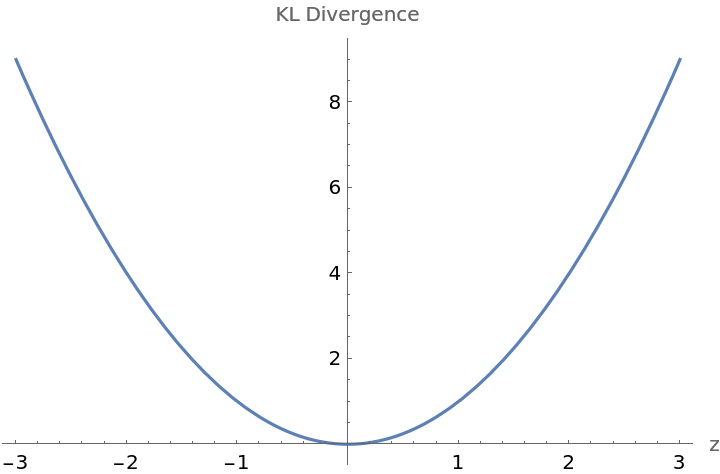### Scope (3)

Compute the Kullback–Leibler divergence of two normal distributions:

 In:=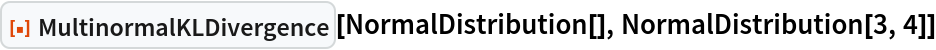Out=Compute the Kullback–Leibler divergence of two binormal distributions:

 In:=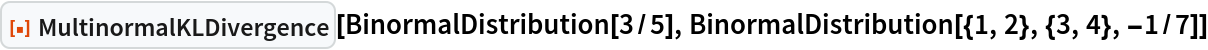Out=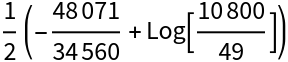Compute the Kullback–Leibler divergence symbolically:

 In:=Out=### Applications (3)

Compare the Kullback–Leibler divergence of two orthogonal multinormal distributions as parameters of their covariance matrices change:

 In:=Out=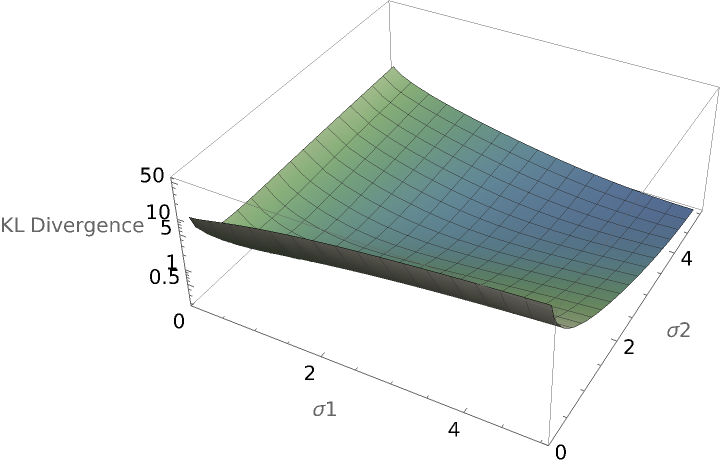Use a Manipulate to compare two multinormal distributions and show their Kullback–Leibler divergence:

 In:=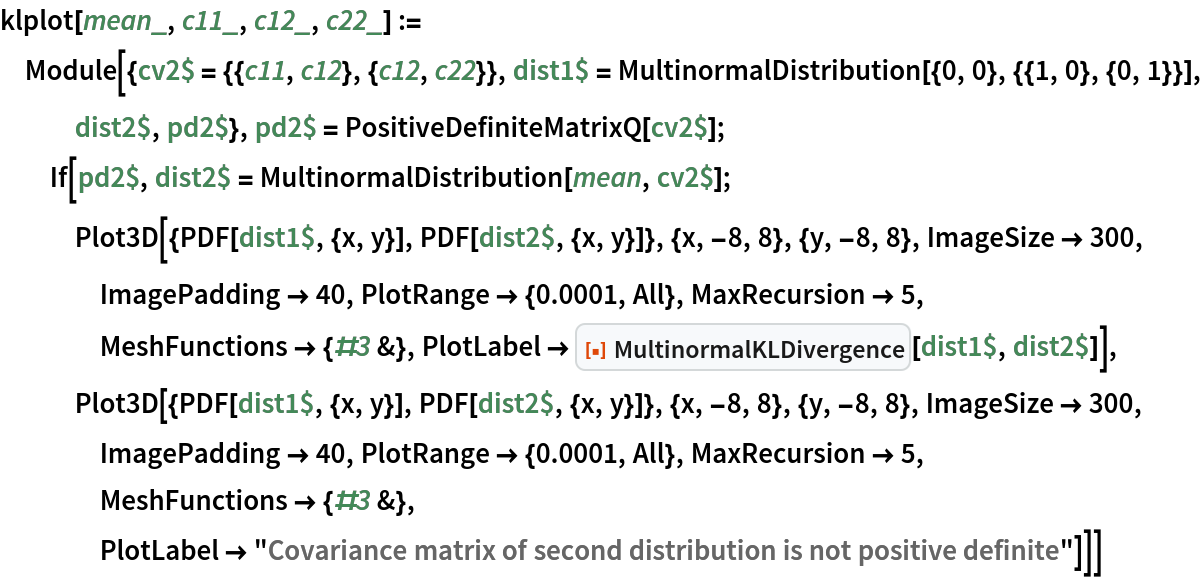In:=Out=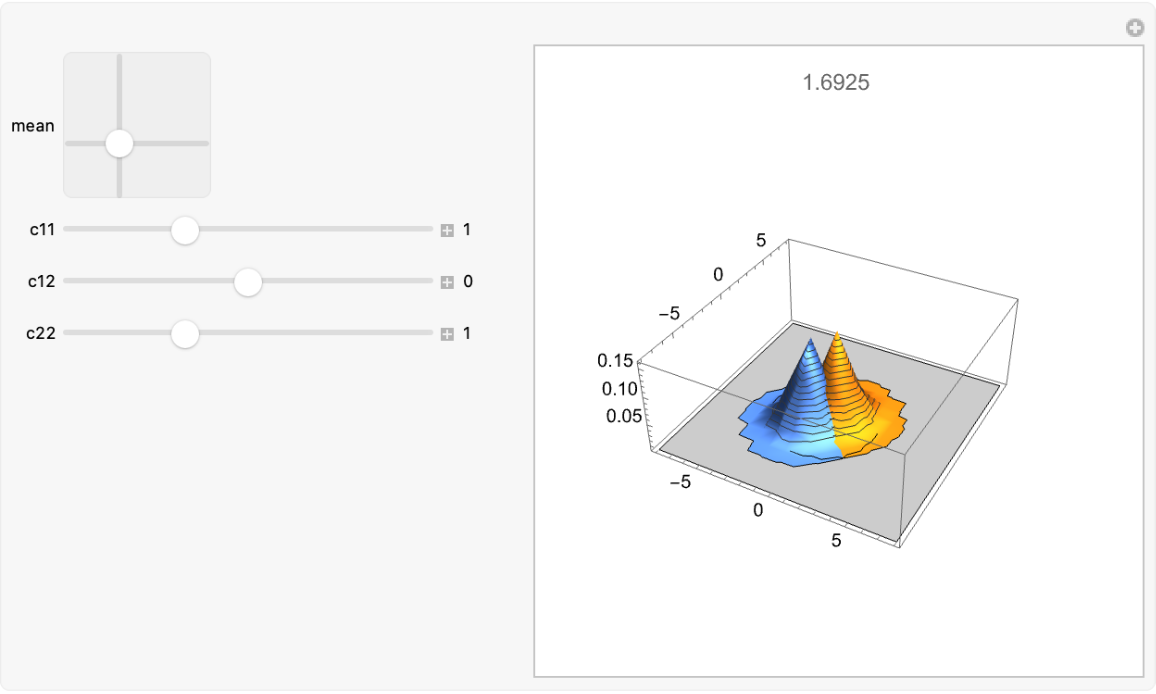Compute the symbolic Kullback–Leibler divergence for a multinormal distribution with itself, but with the second distribution undergoing a rotation of its covariance matrix:

 In:=Out=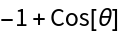The same computation, but with a three dimensional multinormal distribution:

 In:=Out=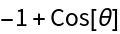### Properties and Relations (2)

One can use the more general resource function KullbackLeiblerDivergence, but MultinormalKLDivergence is somewhat faster for low-dimensional distributions:

 In:=Out=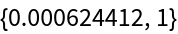In:=Out=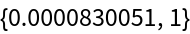MultinormalKLDivergence is much faster for higher-dimensional distributions:

 In:=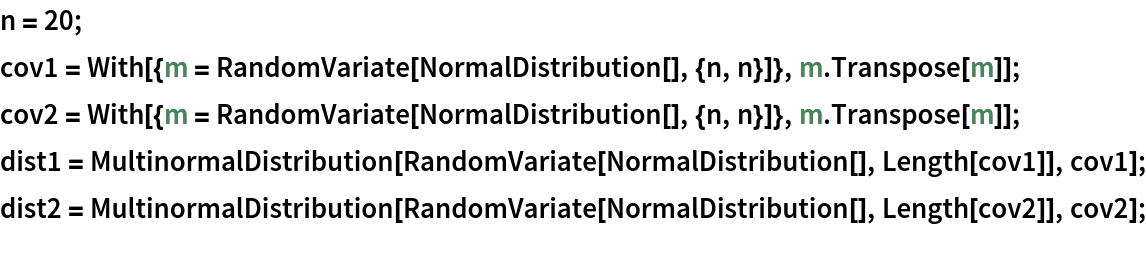In:=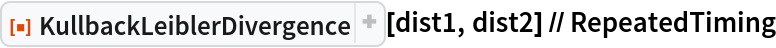Out=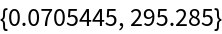In:=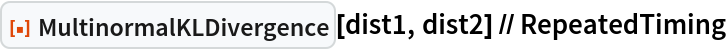Out=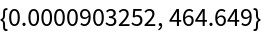### Possible Issues (2)

If the two multinormal distributions supplied have different dimensions, a Failure object will result:

 In:=Out=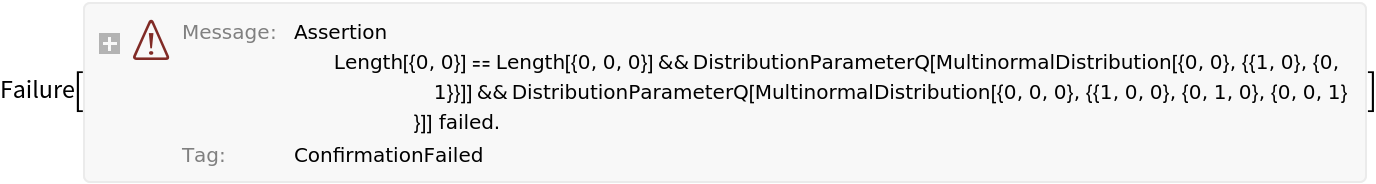If one or more of the distributions is not a multivariate normal distribution, a Failure object will result:

 In:=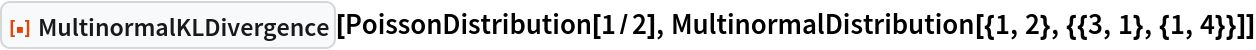Out=### Neat Examples (5)

Use the Kullback–Leibler divergence to help regularization in a variational autoencoder. Create an expression for the Kullback–Leibler divergence between a multinormal distribution with zero mean and a unit, diagonal covariance matrix:

 In:=Out=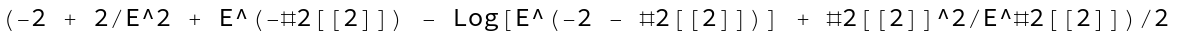Use the resulting expression to create a function layer that can be used for regularization:

 In:=Out=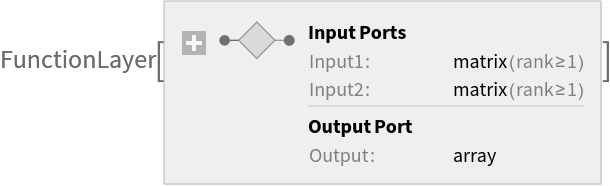Build a variational autoencoder architecture that uses the Kullback–Leibler divergence to prevent overfitting and to encourage the model to learn a compact and meaningful representation of the data:

 In:=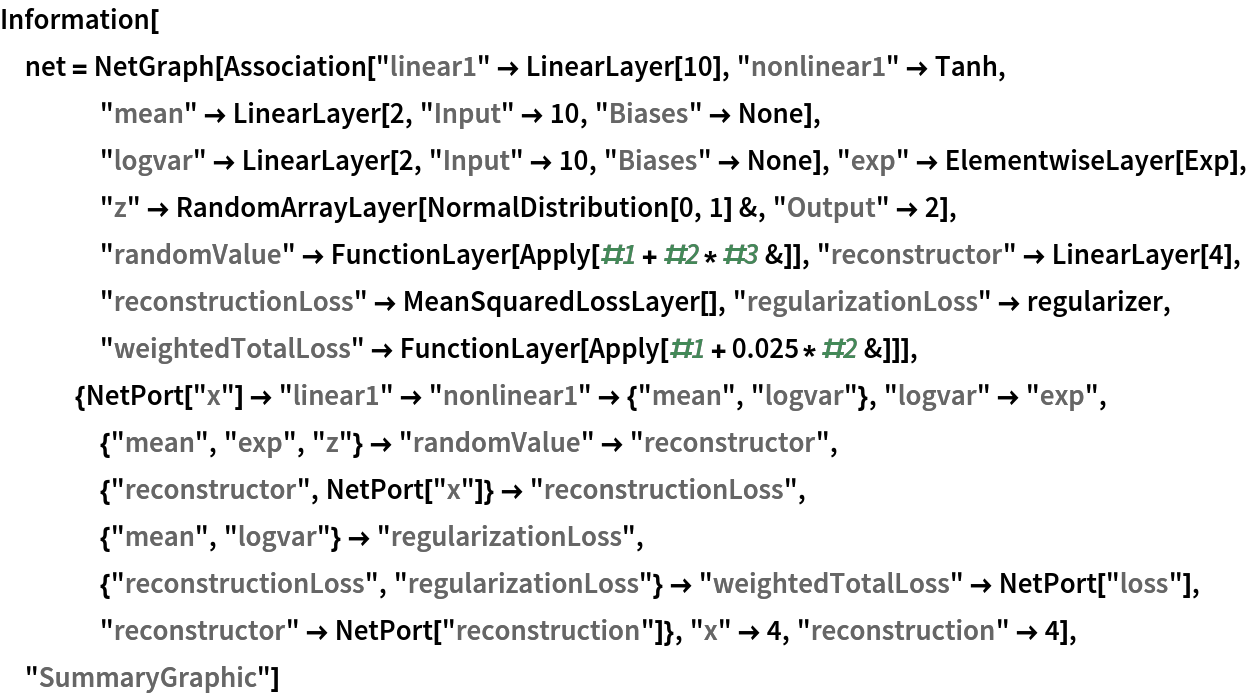Out=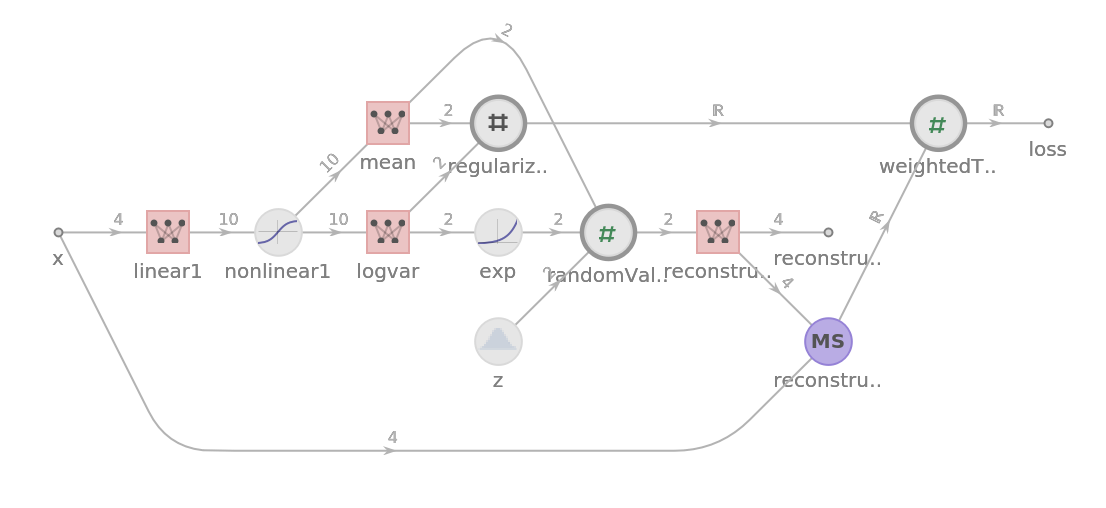Create structured data that one wants the variational autoencoder to capture:

 In:=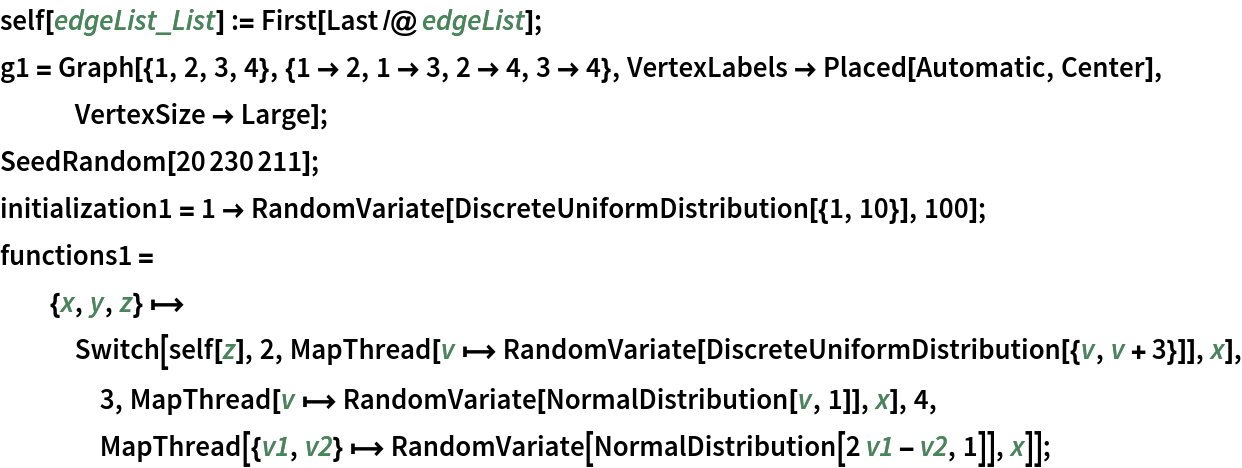In:=Out=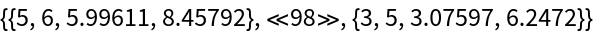In:=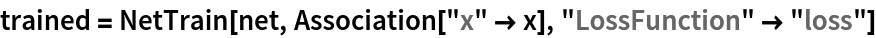Out=Use a RadialAxisPlot to show the smooth and compact reconstruction of the original vector from a latent space:

 In:=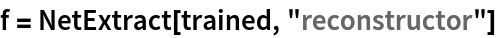Out=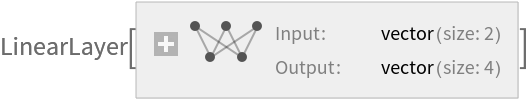In:=Out=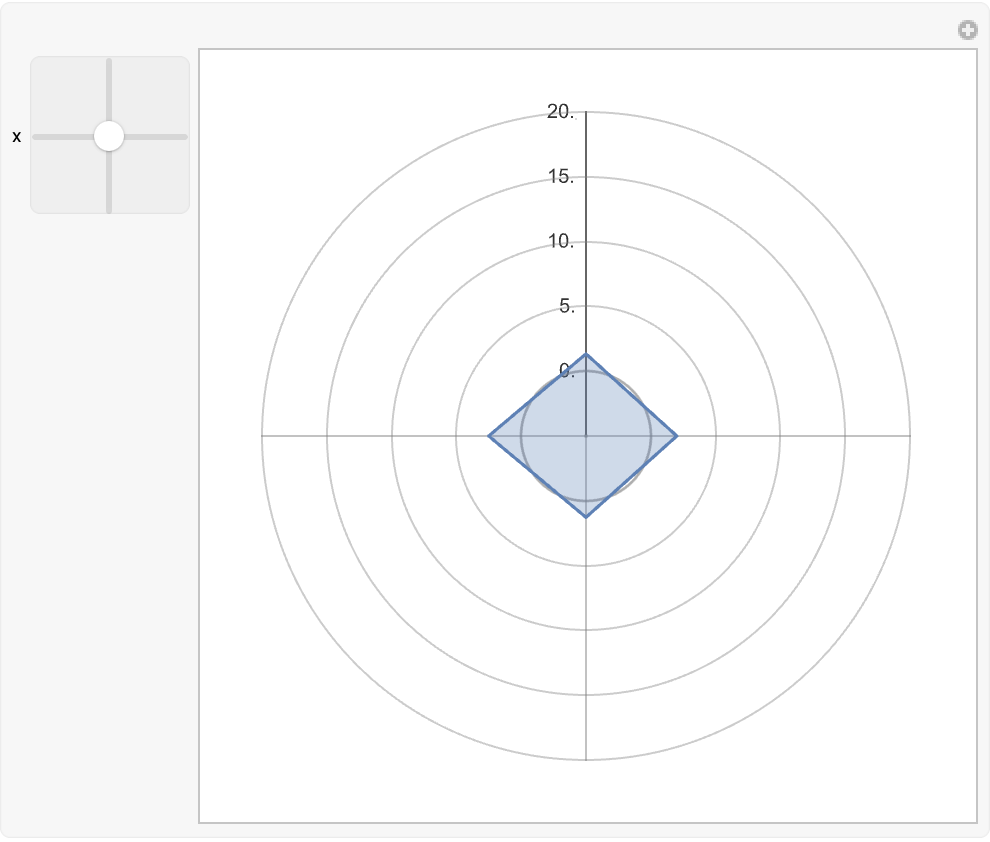Seth J. Chandler

## Version History

• 1.0.0 – 22 February 2023